# Martin Orr's Blog

## Complex abelian varieties and Riemann forms

Posted by Martin Orr on Wednesday, 30 December 2009 at 21:48

The theory of abelian varieties is very beautiful, both in its arithmetic and geometrical aspects, and also looking just over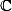where there are nice applications of complex analysis. In this post I will work over, and sketch a proof that a complex torus is isomorphic to an abelian variety if and only if it admits a Riemann form. This will assume some knowledge of the theory of complex manifolds.

### Introduction

An abelian variety is a projective varietywith a multiplication morphismand an inverse morphism, satisfying the usual axioms for a group. It is a surprising fact that the condition thatis projective is enough to guarantee that the multiplication is commutative.

Any abelian variety overis isomorphic (as a complex Lie group) to a complex torus i.e.whereis a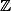-lattice of rank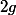in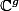. The isomorphism is induced by the exponential map(this is a homomorphism becauseis commutative).

In the case, every complex torusis isomorphic to an elliptic curve. But in higher dimensions, not every complex torus is isomorphic to an abelian variety, because not very complex torus can be embedded in projective space.

### Riemann forms

A Riemann form is an alternating formsuch that

1.for all;
2. the corresponding Hermitian formis positive-definite; and
3.takes integer values on.

Here I am defining a Hermitian form to be a functionwhich is linear in the first variable, and such that.

Ifis a Hermitian form, then the imaginary partis a real alternating form satisfying condition (1) above. Conversely any real alternating form satisfying this identity is the imaginary part of a unique Hermitian form, given by the formula in (2).

(Similarly the real part of a Hermitian form is a symmetric form satisfyingand any such symmetric form is the real part of a unique Hermitian form.)

A Hermitian formis positive-definite iffor all.
In terms of the corresponding alternating form, this is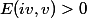for all.

### Hodge metrics

Given a complex torus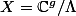, we can viewas the tangent space at the identity. Hence a non-degenerate alternating formongives rise to a 2-formon(which is by definition an alternating form on the tangent space at each point of; we defineatby translatingfrom the identity to).

Sincehas constant coefficients in the natural coordinate charts on, it is a closed 2-form, i.e. a symplectic form.

Conversely, given a translation-invariant symplectic form you can evaluate it at the identity to get a non-degenerate alternating form on. So there is a bijection between non-degenerate alternating forms onand translation-invariant symplectic forms on.

Restricting this to alternating forms which come from a positive-definite Hermitian form, we get a bijection between alternating forms satisfying (1) and (2) above and translation-invariant Kähler metrics on.

Condition (3), namely thattakes integer values on, is equivalent to the corresponding translation-invariant 2-form being in the integral cohomology.

A Kähler metric where the corresponding 2-form is in an integral cohomology class is called a Hodge metric. Hence Riemann forms forbiject with translation-invariant Hodge metrics on.

### Kodaira embedding theorem

Now the fact that any complex torus with a Riemann form is an abelian variety follows immediately from the Kodaira embedding theorem:

Theorem. Any compact complex manifold with a Hodge metric can be embedded in projective space.

The general proof of the Kodaira embedding theorem is hard. Even the dimension 1 case, that any compact Riemann surface is projective, requires some deep analysis.

In the case of complex tori, I believe that it is possible to give an easier proof by explicitly constructing meromorphic functions, using theta functions.

### Other direction

To prove the other direction, that any abelian variety corresponds to a complex torus admitting a Riemann form, is easier.

Given any projective complex manifold, just restrict the Fubini-Study metric onto get a Hodge metric on.

For a complex torus, average this metric over the manifold to get a translation-invariant Hodge metric. We have seen that this corresponds to a Riemann form.

1. Alex Youcis said on Wednesday, 01 October 2014 at 07:44 :

I'm sure you know this, but there is another nice application of complex analysis lurking about. You said "it's a surprising fact that being projective is enough to guarantee commutativity." That said, if A is an abelian variety over C, as in your definition, then A is, in particular, a complex Lie group. Looking at its adjoint representation, this is a map A to GL_n(C) which is holomorphic. Since A is compact, and GL_n(C) affine, this implies that the adjoint representation is trivial, and so A is abelian.

This also works for A any compact complex Lie group, and so it suffices to take A to be proper. But, as I'm sure you know, this was equivalent to projective anyways.

Nice blog by the way. :)